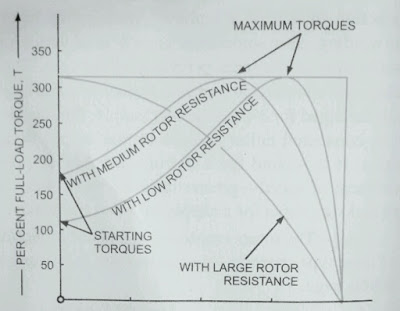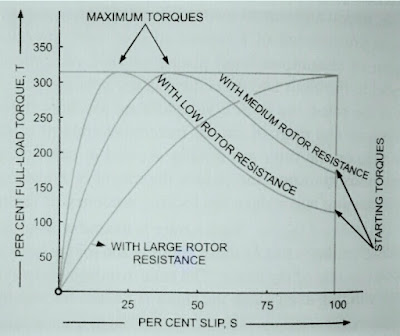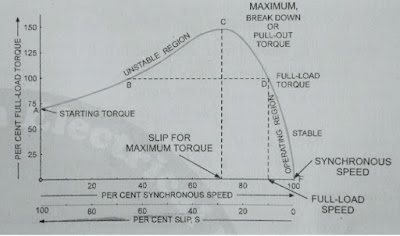### Ticker

6/recent/ticker-posts

# Torque - Slip and Torque - speed Curves of 3 - phase Induction Motor

## Torque - Slip and Torque - Speed Curves

KsR2E²2
From the Eq. T = -------------------  For Torque
R²2 + s²X²2
It Is obvious that

i) at Synchronous speed slip s is zero and so the torque is zero.

ii) at speeds near synchronous speed, the slip is very small, the term sX2 is very small in comperison to R2 and the torque is approximately Proportional to slip s.Fig. (1) Torque - Speed Curves

iii) with the increase in slip, speed decrease, torque Increases and reach.Fig. (2) Torque Slip curves

R2
it's maximum value when s = ------- .
X2
The maximum is Torque also known as breakdown or pull out torque.

iv) with the further increase in slip or decrease in speed due to increase in load beyond the point of breakdown or pull - out the torque decrease causing slow down of motor.

The motor will eventually stop.

v) With higher slip R2 becomes negligible in comperison to sX2 and the torque varies as

1
---- i.e., torque speed or Torque slip Curves
s

Rectangular hyperbola with the slip or sleep beyond pull out or breakdown point.

These Curves are shown in fig. 1 and 2 respectively.

It is seen that although maximum torque in Independent of rotor resistance R2, yet the exact location of  T max depends upon rotor resistance R2.

Greater the value of R2, greater is the value of slip at which the maximum torque occurs.

### Effect of Rotor Resistance upon Torque. Slip or Torque - speed Relationship.

For a given value of torque T, slip s is Proportional to rotor resistance R2, So addition of external resistance in the rotor Circuit does not Lower the torque curve but merely stretches it so that the same torque values occur at lower speed (or Higher slip)  as shown in figs 1 and 2.

### Full Load Torque and Maximum Torque.

Ratio of full Load Torque and Maximum Torque is given by relationship

Tf                    2
-----------  =  ---------------------      ..... ( 1 )
T max       s max T      s f
-------  + ---------
sf        s max T

At starting.  sf = 1 and so we have

T st            2a
---------  =  --------                    .....( 2 )
T max     a² + 1

R2
Where a = ------
X2

### Torque Speed Curve and Operating Region.

The torque - speed Curve for an Induction Motor is given in fig 3.

From fig.3 it is evident that for any load torque, there are two operating points (say B and D ). But at position B, the Operation is unstable because if there is tendency of speed rise the developed torque also increase than the load torque causing further rise in speed. Thus at position B the operation is unstable.

At position D operation is stable because a tendency to rise in speed will be opposed by the decrease in development torque similarly when there is tendency to fall in speed , there will be increased a developed torque to bring the motor to operating position D.Fig. (3)

Thus the region AC of the torque - speed characteristics is unstable region of operation while the region CF is stable region of operation.

At full load the motor runs at a speed of N rpm.

If the Mechanical load applied to the motor is increased , motor speed drops till the torque developed by the motor equals the load torque.

As long as the two torque are in balance, the motor will run at constant speed. However, if the load torque exceeds the breakdown torque of the motor, the motor will immediately stop.

The value of breakdown torque varies with the design of the motor but ranges from 200 to 300 percent of full load torque in Standard Squirrel cage Motors.

The torque speed characteristics of an Induction Motor in the operating region are quite similar to those of a DC shunt motor.

Even at full load, the drop speed from no - load does not exceed 5 percent.